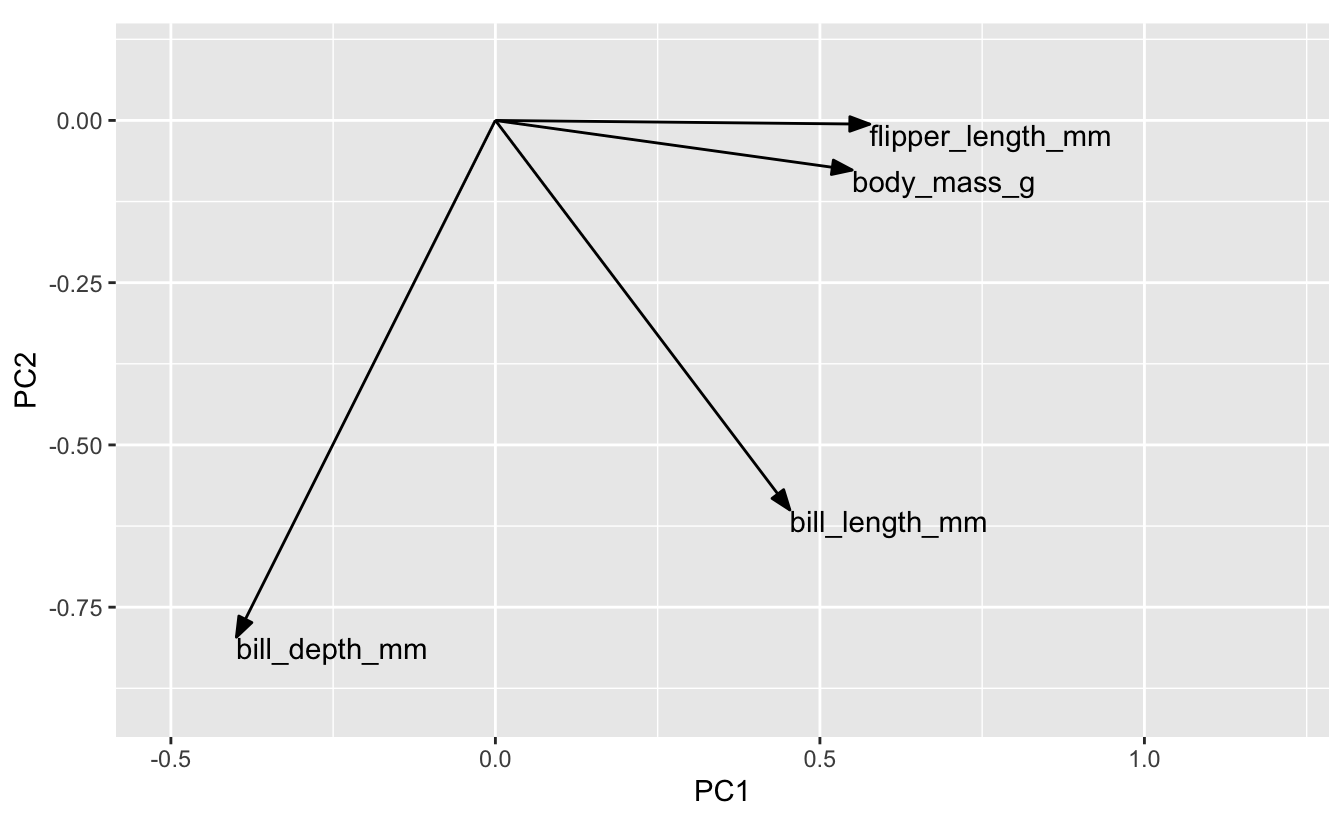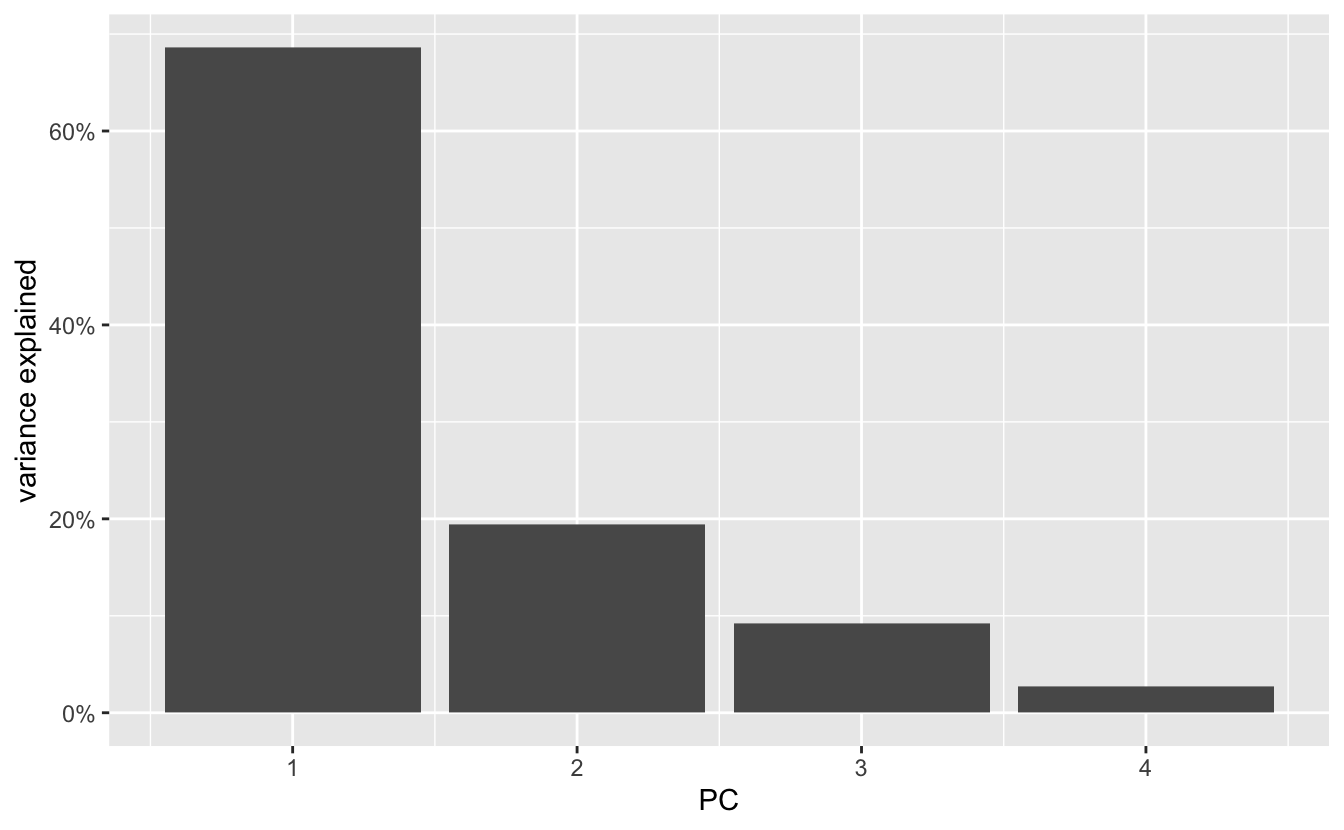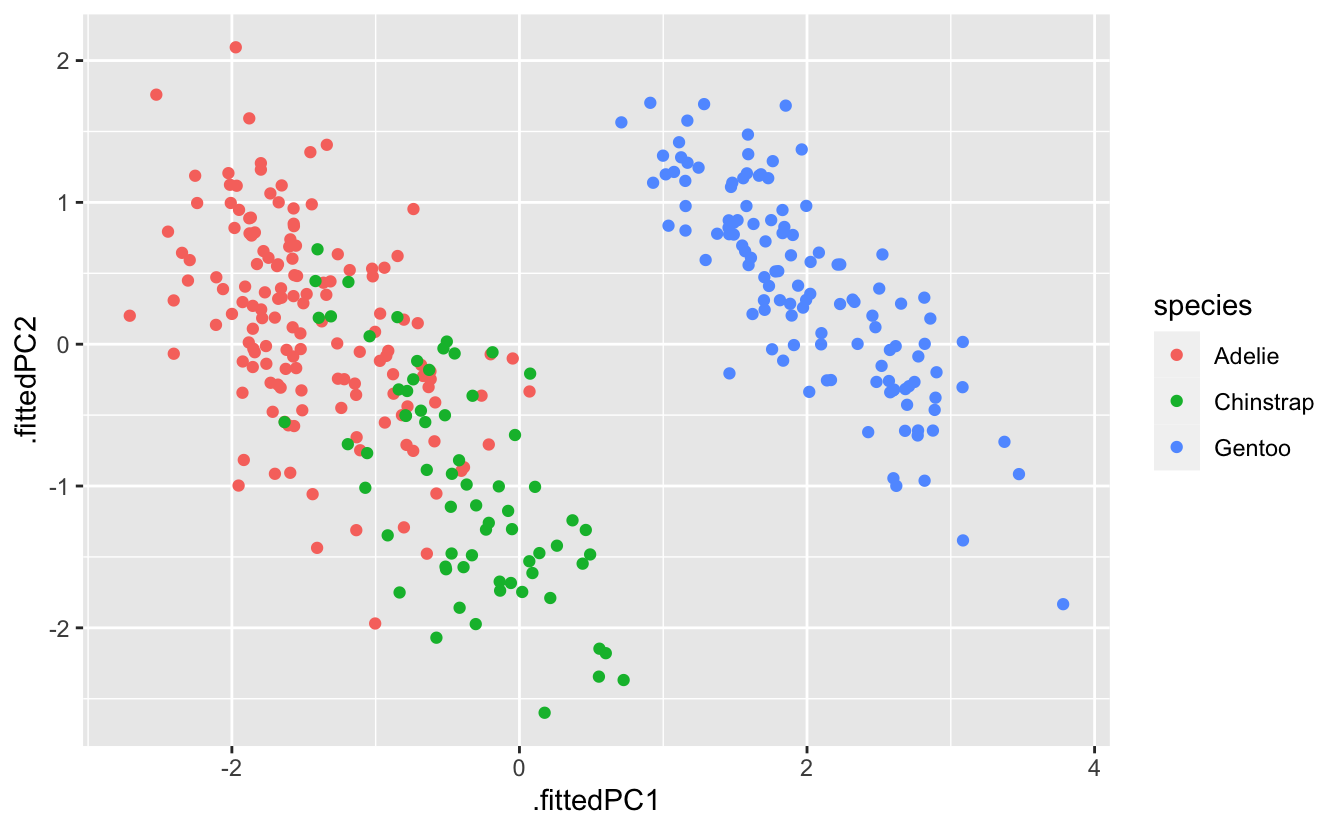Enter your name and EID here

This homework is due on April 12, 2021 at 11:00pm. Please submit as a pdf file on Canvas.

For all problems in this homework, we will work with the `penguins_clean` dataset, which is a cleaned-up version of the `penguins` dataset from the palmerpenguins package.

Note: This homework is about the contents of the plots. Don’t worry about styling. It’s OK to use the default theme and plot labeling.

``````library(palmerpenguins)

penguins_clean <- penguins %>%
select(-year) %>% # remove the year column as it is distracting here
na.omit()         # remove any rows with missing values

penguins_clean``````
``````## # A tibble: 333 x 7
##    species island bill_length_mm bill_depth_mm flipper_length_… body_mass_g
##    <fct>   <fct>           <dbl>         <dbl>            <int>       <int>
##  1 Adelie  Torge…           39.1          18.7              181        3750
##  2 Adelie  Torge…           39.5          17.4              186        3800
##  3 Adelie  Torge…           40.3          18                195        3250
##  4 Adelie  Torge…           36.7          19.3              193        3450
##  5 Adelie  Torge…           39.3          20.6              190        3650
##  6 Adelie  Torge…           38.9          17.8              181        3625
##  7 Adelie  Torge…           39.2          19.6              195        4675
##  8 Adelie  Torge…           41.1          17.6              182        3200
##  9 Adelie  Torge…           38.6          21.2              191        3800
## 10 Adelie  Torge…           34.6          21.1              198        4400
## # … with 323 more rows, and 1 more variable: sex <fct>``````

Problem 1: (2 pts)

Perform a PCA of the `penguins_clean` dataset and make two plots: 1. A rotation plot of components 1 and 2; 2. A plot of the eigenvalues, showing the amount of variance explained by the various components.

``````# perform PCA
pca_fit <- penguins_clean %>%
select(where(is.numeric)) %>% # retain only numeric columns
scale() %>%                   # scale to zero mean and unit variance
prcomp()

# make rotation plot
arrow_style <- arrow(
angle = 20, length = grid::unit(8, "pt"),
ends = "first", type = "closed"
)
pca_fit %>%
# extract rotation matrix
tidy(matrix = "rotation") %>%
pivot_wider(
names_from = "PC", values_from = "value",
names_prefix = "PC"
) %>%
ggplot(aes(PC1, PC2)) +
geom_segment(
xend = 0, yend = 0,
arrow = arrow_style
) +
geom_text(aes(label = column), hjust = 0, vjust = 1) +
coord_fixed(xlim = c(-0.5, 1.2), ylim = c(-.9, .1))````````````# make variance explained plot
pca_fit %>%
# extract eigenvalues
tidy(matrix = "eigenvalues") %>%
ggplot(aes(PC, percent)) +
geom_col() +
scale_x_continuous(
# create one axis tick per PC
breaks = 1:4
) +
scale_y_continuous(
name = "variance explained",
# format y axis ticks as percent values
label = scales::label_percent(accuracy = 1)
)``````Problem 2: (4 pts) Make a scatter plot of PC 2 versus PC 1 and color by penguin species. Then use the rotation plot from Problem 1 to describe the physical characteristics by which the different penguin species differ. Finally, make one more scatter plot of the raw data that can support your interpretation of the PC analysis.

``````pca_fit %>%
# add PCs to the original dataset
augment(penguins_clean) %>%
ggplot(aes(.fittedPC1, .fittedPC2)) +
geom_point(aes(color = species))``````All three species separate along PC 1. Penguins with high PC 1 have on average higher body mass, longer flippers, and longer but narrower bills. It approximately represents the overall size of the penguins. Therefore, Gentoo penguins are substantially larger than the other two species. However, Chinstrap don’t differ from Adelie in body mass. Instead, they have longer bills.

Supporting plot:

``````penguins_clean %>%
ggplot(aes(body_mass_g, bill_length_mm)) +
geom_point(aes(color = species))``````